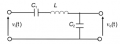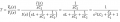# Inverse Laplace transform

Thread Starter

#### mojo_risin

Joined Jul 3, 2013
35
Hello
I am deriving the transfer function of the circuit below:I have determined it to be 1/[(s^2*L*C2)+(C2/C1)+1]. I have values for the elements as follows: C1 = 0.1 F, C2 = 0.01F, L = 0.5H. When I substitute these values in the transfer function, I have the expression
T = 200/(s^2 + 220). I do not know how to simplify it so that I would be able to take the inverse Laplace and convert it to time domain; been trying all day long now. Here is what I have:Please share any suggestions. Thank you.

#### Papabravo

Joined Feb 24, 2006
16,072
Can you use a partial fraction expansion?

Thread Starter

#### mojo_risin

Joined Jul 3, 2013
35
I can't think of suitable choice of s, that will eliminate one of the constants. If I choose two arbitrary constants for s and solve the resulting equations, I get a messy answer that I do not want to post, but is clearly leading me to nowhere. Just out of curiosity, are you able to evaluate the expression?

#### Papabravo

Joined Feb 24, 2006
16,072
Yes I can do it. You need to reread the section on how to eliminate one of the terms by a suitable multiplication followed by an evaluation of the remaining term for a particular value of s. If the link I gave you is not crystal clear then look for another that explains partial fraction expansion.

#### Papabravo

Joined Feb 24, 2006
16,072
The original factorization of the denominator is wrong. You obviously have not read the text associated with the method because you keep trying to do it the same wrong way.
Code:
Let T(s) = 2 /(s+1)(s+2) = A/(s+1) + B/(s+2)
*** Step 1 ***
*** MULTIPLY BOTH SIDES by (s+1) ***
2/(s+2) = A + B(s+1)/(s+2)
*** NOTICE how coefficient A has been isolated all alone by itself! ***
*** Now -- EVALUATE what is left at s = -1 ***
2/(-1 + 2) = A + B(-1 +1)/(-1+2)
2 = A
*** End of Step 1 ***
*** Step 2 ***
*** MULTIPLY BOTH SIDES by (s+2) ***
2/(s+1) = A(s+2)/(s+1) + B
*** NOTICE how coefficient B has been isolated all alone by itself! ***
*** Now -- EVALUATE what is left at s = -2 ***
-2 = B
*** End of Step 2 ***
That was the case for the roots of the denominator being real and distinct.
There are of course more cases. In your case the roots of the denominator are a complex conjugate pair lying on the imaginary axis. The details are slightly different but the method remains the same. Are you seriously trying to tell us that you missed completely the idea of multiplying both sides by one of the factors of the denominator?

Thread Starter

#### mojo_risin

Joined Jul 3, 2013
35
Just noticed that the factorization is wrong. That's to be expected.
I am going to go back and check all the work that led me to that transfer function.

#### Papabravo

Joined Feb 24, 2006
16,072
That's a good thing. Did you understand the two basic steps in doing partial fraction expansions? Can you generalize the method to the other cases of roots for the characteristic equation? It is my hope that by the time we are done you will know this stuff like the back of your hand. Just for reference the cases are:
1. Roots of the denominator of T(s) are real and distinct
2. Roots of the denominator of T(s) are real and repeated
3. Roots of the denominator of T(s) are complex or imaginary

Last edited:
Thread Starter

#### mojo_risin

Joined Jul 3, 2013
35
Yes, I did. Sometimes when I get on the wrong track I keep going and going. Bad trait. It's my first time dealing with expression that has complex roots. I believe I have done something wrong while deriving the function in the first place.

#### Papabravo

Joined Feb 24, 2006
16,072
OK, so let us know what you figure out and if there are other questions that we can help you with.

#### MrAl

Joined Jun 17, 2014
8,231
Hello,

Are you are trying to get the impulse response in the end?

Similar threads